# Gibbs sampling for the two-sample t-test

### Chapter 3.2.1: Gibbs sampling

In this exercise we will use Gibbs sampling to test whether two populations have the same mean. Let the data from the first population be $Y_1,…,Y_n\sim\mbox{Normal}(\mu_Y,\sigma^2)$ and the second population be $Z_1,…,Z_m\sim\mbox{Normal}(\mu_Z,\sigma^2).$ The priors are $$\mu_Y,\mu_Z\sim\mbox{Normal}(\mu_0,\sigma_0^2)$$ and $$\sigma^2\sim\mbox{InvGamma}(a,b)$$.

Our goal is to compute the posterior of $$\Delta=\mu_Y-\mu_Z$$ and determine if $$\Delta=0$$ (the populations have the same mean) or not. To do these, we compute $$\Delta$$ at each iteration and then compute the posterior 95% credible set. To test out the code we will simulate data so we know the true values.

## Simulate data

 set.seed(1)

n         <- 20
m         <- 20
muY_true  <- 55
muZ_true  <- 50
sig2_true <- 100

Y <- rnorm(n,muY_true,sqrt(sig2_true))
Z <- rnorm(n,muZ_true,sqrt(sig2_true))

boxplot(cbind(Y,Z))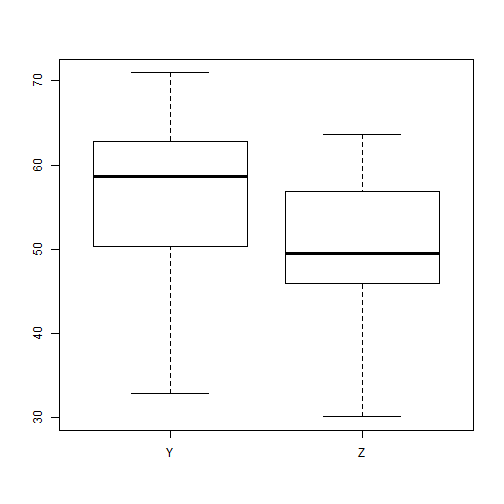## Set the priors

  mu0 <- 0
s20 <- 1000
a   <- 0.01
b   <- 0.01


## Gibbs sampling

  n.iters <- 30000
keepers <- matrix(0,n.iters,4)
colnames(keepers)<-c("muY","muZ","sigma2","Delta")

# Initial values
muY         <- mean(Y)
muZ         <- mean(Z)
s2          <- (var(Y)+var(Z))/2
keepers[1,] <- c(muY,muZ,s2,muY-muZ)

for(iter in 2:n.iters){

# sample muY|muZ,s2,Y,Z

A   <- sum(Y)/s2+mu0/s20
B   <- n/s2+1/s20
muY <- rnorm(1,A/B,1/sqrt(B))

# sample muZ|muY,s2,Y,Z

A   <- sum(Z)/s2+mu0/s20
B   <- m/s2+1/s20
muZ <- rnorm(1,A/B,1/sqrt(B))

# sample s2|muY,muZ,Y,Z

A  <- n/2+m/2+a
B  <- sum((Y-muY)^2)/2 + sum((Z-muZ)^2)/2+b
s2 <- 1/rgamma(1,A,B)

# keep track of the results
keepers[iter,] <- c(muY,muZ,s2,muY-muZ)

}


## Plot convergence

 plot(keepers[,1],type="l",ylab="muY")
abline(muY_true,0,col=2,lwd=2)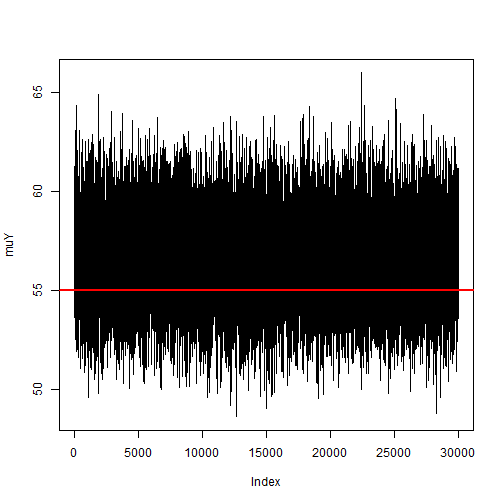plot(keepers[,2],type="l",ylab="muZ")
abline(muZ_true,0,col=2,lwd=2)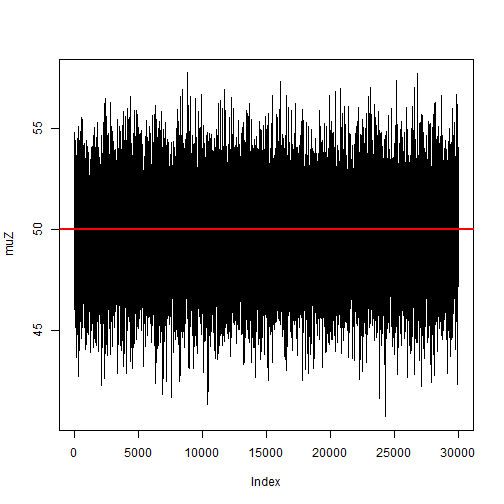plot(keepers[,3],type="l",ylab="s2")
abline(sig2_true,0,col=2,lwd=2)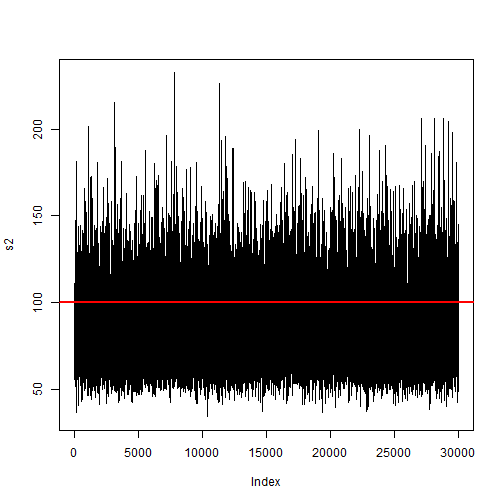plot(keepers[,4],type="l",ylab="Delta")
abline(muY_true-muZ_true,0,col=2,lwd=2)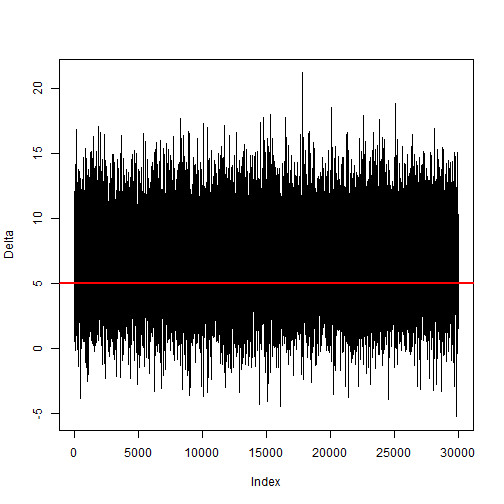## Plot the joint posterior

 pairs(keepers)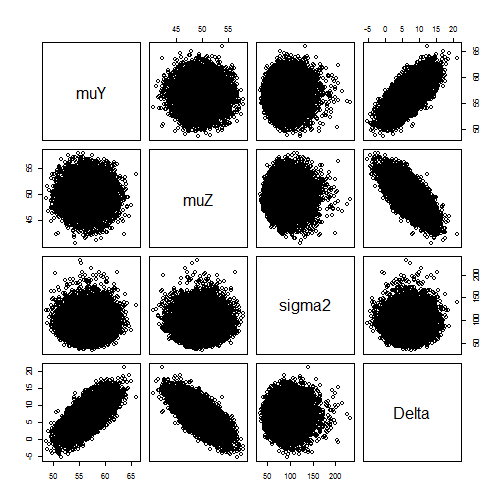## Summarize the marginal posterior of $$\Delta=\mu_Y-\mu_Z$$, $$p(\Delta|Y,Z)$$

 Delta <- keepers[,4]
hist(Delta,main="Posterior of the difference in means",breaks=100)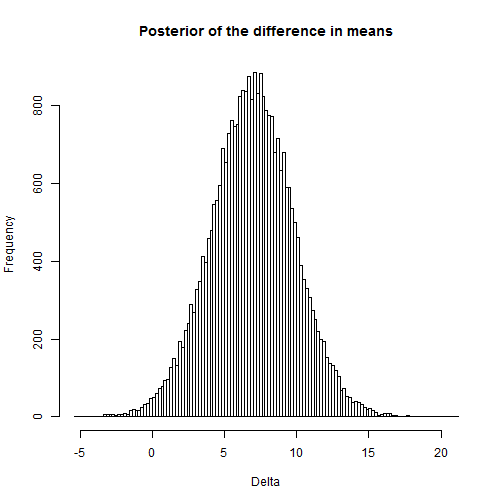muY_true;muZ_true;muY_true-muZ_true # True values

##  55

##  50

##  5

 quantile(Delta,c(0.025,0.975)) # Posterior 95% credible set

##      2.5%     97.5%
##  1.263061 12.612540

 mean(Delta>0) # Posterior probibility that muY>muZ

##  0.9911667